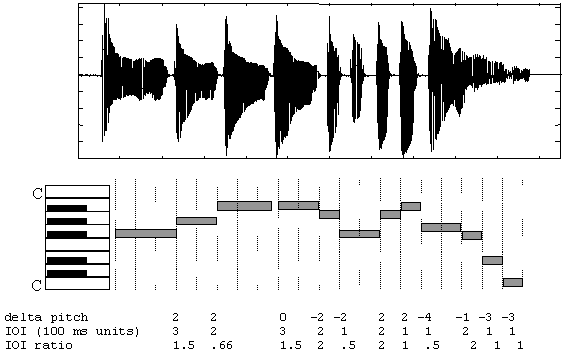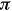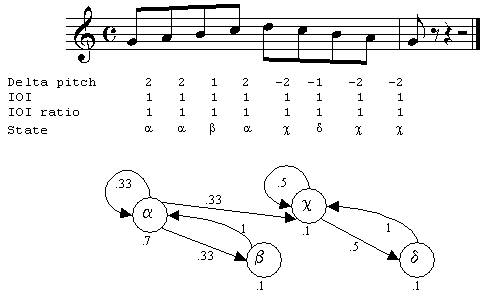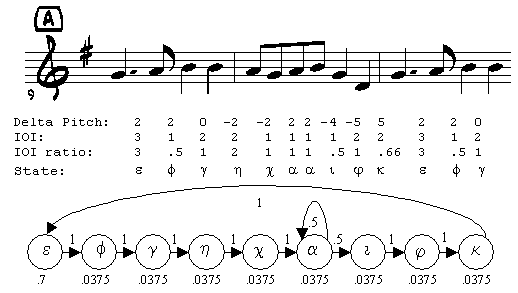## The MusArt Music-Retrieval System

### Appendix 1: The Hidden Markov Model

William Birmingham, Bryon Pardo, Colin Meek, and Jonah Shifrin
Department of Electrical Engineering and Computer Science
The University of Michigan

#### Representation of a Query

As shown in Figure 1, the user's query is transcribed by a pitch tracker into a form usable by MusArt. The query is recorded as a .wav file and then transcribed into a MIDI1-based representation using an enhanced autocorrelation algorithm .Figure 1: MusArt Architecture

MIDI is to a digital audio recording of music as ASCII is to a bitmap image of a page of text. Note events in MIDI are specified by three integer values in the range 0 to 127. The first value describes the event type (e.g. "note off" and "note on"). The next value specifies which key on a musical keyboard was depressed. Generally, middle "C" gets the number 60. The final integer specifies the velocity of a note (used as an indication of loudness). Pitch tracking can be thought of as the equivalent of character recognition in the text world.Figure 1A: A sung query

The pitch tracker divides the input file into 10 millisecond frames and tracks pitch on a frame-by-frame basis. Contiguous regions of at least five frames (50 millisecond) whose pitch varies less than one musical half step are called notes. The pitch of each note is the average of the pitches of the frames within the note. The pitch tracker returns a sequence of notes, each of which is defined by pitch, onset time and duration. Pitches are quantized to the nearest musical half step and represented as MIDI pitch numbers.

Figure 1A shows a time-amplitude representation of a sung query, along with example pitch-tracker output (shown as piano roll) and a sequence of values derived from the MIDI representation (the deltaPitch, IOI and IOIratio values). Time values in the figure are rounded to the nearest 100 milliseconds.

We define the following.

• A note transition between note n and note n+1 is described by the duple <deltaPitch, IOIratio>.
• deltaPitchn is the difference in pitch between note n and note n+1.
• The inter onset interval (IOIn) is the difference between the onset of notes n and n+1.
• IOIration is IOIn/IOIn+1. For the final transition, IOIn = IOIn /durationn+1.

We represent a query as a sequence of note transitions. Note transitions are useful because they are robust in the face of transposition and tempo changes. The deltaPitch component of a note transition captures pitch-change information. Two versions of a piece played in two different keys have the same deltaPitch values. The IOIratio represents the rhythmic component of a piece. This remains constant even when two performances are played at very different speeds, as long as relative durations within each performance remain the same.

In order to reduce the number of possible IOI ratios, we quantize them to a logarithmic scale within a fixed range. A logarithmic scale was selected because data from a pilot study indicated that sung IOIratio values fall naturally into evenly spaced bins in the log domain.

#### Markov Representation

A Markov model (MM) models a process that goes through a sequence of discrete states, such as notes in a melody. The model is a weighted automaton that consists of:

• A set of states, S={s1, s2, s3,, sn}.
• A set of transition probabilities between states, T.
• A probability distribution,whereis the probability the automaton will begin in state si.
• E, a subset of S containing the legal ending states.

In this model, the probability of transitioning from a given state to another state is assumed to depend only on the current state. This is known as the Markov property.

The directed graph in Figure 2A represents a Markov model of a scalar passage of music. States are note transitions. Nodes represent states. The numerical value below each state indicates the probability a traversal of the graph will begin in that state. As a default, we currently assume all states are legal ending states. Directed edges represent transitions. Numerical values by edges indicate transition probabilities. Only transitions with non-zero probabilities are shown.

Here, we have implicitly assumed that whenever state s is reached, it is directly observable, with no chance for error. This is often not a realistic assumption, as we noted earlier, there are multiple possible sources of error in generating a query. Such errors can be handled gracefully if a probability distribution over the set of possible observations (such as note transitions in a query) given a state (the intended note transition of the singer) is maintained.Figure 2A: Markov model for a scalar passage

A model that explicitly maintains a probability distribution over the set of possible observations for each state is called a hidden Markov model (HMM). More formally, an HMM requires two things in addition to that required for a standard Markov model:

• A set of possible observations, O={o1, o2, o3,, on}.
• A probability distribution over the set of observations for each state in S.

In our approach, a query is a sequence of observations. Each observation is a note-transition duple, <deltaPitch, IOIratio>. Musical themes are represented as hidden Markov models.

#### Making Markov Models from MIDI

Our system represents musical themes in a database as HMMs. The unique duples characterizing the note transitions found in the MIDI file form the states in the model. Figure 3A shows a passage with eight note transitions characterized by four unique duples. Each unique duple is represented as a state.

Once the states are determined for the model, transition probabilities between states are computed by calculating what proportion of the time state a follows state b in the theme. Often, this results in a large number of deterministic transitions. Figure 3A is an example of this, where only a single state has two possible transitions, one back to itself and the other on to the next state. The calculation for initial state distributions is handled in a special way, and is not discussed in this paper.Figure 3A: Markov model for Alouette fragment

Markov models can be thought of as generative models. A generative model describes an underlying structure able to generate the sequence of observed events, called an observation sequence.

Note that there is not a one-to-one correspondence between model and observation sequence. A single model may create a variety of observation sequences, and an observation sequence may be generated by more than one model. Recall that our approach defines an observation as a duple, <deltaPitch, IOIratio>. Given this, the observation sequence q = {(2,1), (2,1), (2,1)} may be generated by the HMM in Figure 2A or the HMM in Figure 3A.

#### Estimating Observation Probabilities

To make use of the strengths of a hidden Markov model, it is important to model the probability of each observation oi in the set of possible observations, O, given a hidden state, s.

Observations consist of duples <deltaPitch, IOIratio>. There are twelve musical half steps in an octave. If one assumes pitch quantized at the half step and that a singer will jump by no more than an octave between notes, there are 25 possible deltaPitch values. We quantize IOIratio to one of 27 values. This means there are 25*27= 675 possible observations given a hidden state. Since hidden states are also characterized by <deltaPitch, IOIratio>, there are 675 possible hidden states for whom observation probabilities need to be determined. The resulting table has 6752, or over 450,000 entries.

We use a typical method to estimate probabilities . A number of observation-hidden state pairs are collected into a training set and observed frequencies are used as an estimator for expected probabilities. Given a state, s, the probability of observation oi may be estimated by the count of how often oi is seen in state s, compared to the total number of times s is entered.

Training our HMM is a difficult task, given the size of the state space. To make the task tractable, we assume that duration errors and pitch errors are conditionally independent. This greatly reduces the number of training cases we need; however, there will be observations that do not occur in the training data. Conditional independence allows us to induce values for the aforementioned 450,000-entry table from the values in two considerably smaller tables; for deltaPitch, we need only consider 25*25 = 625 hidden state/observation pairs, and for IOIratio only 27*27 = 729 pairs.

We cannot assume that a pairing unobserved in the training set will never be observed in an actual query. Thus, we impose a minimal probability, Pmin (typically a value close to zero), on both observation probability tables. Any probability falling below Pmin is set to Pmin. Probabilities are then normalized so that the sum of all observation probabilities, given a particular hidden state, is again equal to one.

#### Notes and References

 Musical Instrument Digital Interface (MIDI) is a standard representation for musical performances or scores.

 Mazzoni, D. and R.B. Dannenberg. Melody Matching Directly from Audio. in ISMIR. 2001.

 R. Durbin, S.E., A. Krogh, G. Mitchison, Biological Sequence Analysis: Probabilistic models of proteins and nucleic acids. 1998, Cambridge, UK: Cambridge University Press.

###### Copyright© 2002 William Birmingham, Bryan Pardo, Colin Meek, and Jonah Shifrin.